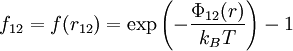# Mayer f-function$f_{12}=f(r_{12})= \exp\left(-\frac{\Phi_{12}(r)}{k_BT}\right) -1$
•$k_B$ is the Boltzmann constant.
•$T$ is the temperature.
•$\Phi_{12}(r)$ is the intermolecular pair potential.